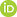Full Text:<2211>

Summary:<1773>

CLC number: TU452

On-line Access: 2015-11-04

Revision Accepted: 2015-07-17

Crosschecked: 2015-10-12

Cited: 0

Clicked: 4595

Citations:  Bibtex RefMan EndNote GB/T7714ORCID:

Yong Zhang

http://orcid.org/0000-0003-3248-5463

### -   Go to

Article info.
 Journal of Zhejiang University SCIENCE A 2015 Vol.16 No.11 P.922-934 http://doi.org/10.1631/jzus.A1400364A semi-analytical method and its application for calculating the thermal stress and displacement of sparsely fractured rocks with water flow and heat transfer

 Author(s):  Yong Zhang, Yan-yong Xiang Affiliation(s):  1School of Civil Engineering, Beijing Jiaotong University, Beijing 100044, China; more Corresponding email(s):   xiang_yanyong@263.net Key Words:  Sparsely fractured rock, Water flow, Heat transfer, Thermo-elastic displacement potential, Thermal stress Share this article to： More <<< Previous Article|

Yong Zhang, Yan-yong Xiang. A semi-analytical method and its application for calculating the thermal stress and displacement of sparsely fractured rocks with water flow and heat transfer[J]. Journal of Zhejiang University Science A, 2015, 16(11): 922-934.

@article{title="A semi-analytical method and its application for calculating the thermal stress and displacement of sparsely fractured rocks with water flow and heat transfer",
author="Yong Zhang, Yan-yong Xiang",
journal="Journal of Zhejiang University Science A",
volume="16",
number="11",
pages="922-934",
year="2015",
publisher="Zhejiang University Press & Springer",
doi="10.1631/jzus.A1400364"
}

%0 Journal Article
%T A semi-analytical method and its application for calculating the thermal stress and displacement of sparsely fractured rocks with water flow and heat transfer
%A Yong Zhang
%A Yan-yong Xiang
%J Journal of Zhejiang University SCIENCE A
%V 16
%N 11
%P 922-934
%@ 1673-565X
%D 2015
%I Zhejiang University Press & Springer
%DOI 10.1631/jzus.A1400364

TY - JOUR
T1 - A semi-analytical method and its application for calculating the thermal stress and displacement of sparsely fractured rocks with water flow and heat transfer
A1 - Yong Zhang
A1 - Yan-yong Xiang
J0 - Journal of Zhejiang University Science A
VL - 16
IS - 11
SP - 922
EP - 934
%@ 1673-565X
Y1 - 2015
PB - Zhejiang University Press & Springer
ER -
DOI - 10.1631/jzus.A1400364

Abstract:
Using Goodier’s thermo-elastic displacement potential and Laplace transform, a semi-analytical method is developed for calculating the displacement and stress induced by heat transfer in sparsely fractured granitic rocks with saturated water flow and distributed heat sources. An integral equation of the thermo-elastic displacement potential is formulated in the Laplace-transformed domain. The fractures are discretized into rectangular elements, and the elemental integrals that involve singularities are calculated analytically. The numerical solutions of the potential are calculated using numerical Laplace inversion, and the temperature-gradient-induced displacements and stresses are calculated using central differences. The method is employed to examine the characteristics of the temperature-gradient-induced displacement and stress for a hypothetical problem that is intended to mimic the near-field environment of deep geological repositories of high-level radioactive wastes. Among other things, the results reveal the following: (1) In early time of operation of the repository, the region of rock under thermal expansion and compressive is limited; (2) As the intensity of the heat source gets smaller with time, only a small portion of the rock expands whereas the remaining portion contracts; (3) Downstream peak temperatures may be higher due to the supply of thermal energy by the water-flow-facilitated heat transfer, and patterns of influences of the water velocities on the thermal stress and displacement are similar; (4) Sufficiently close heat sources would cause superposition of the heating effects and make the near-field temperature increase significantly.

The manuscript is for calculating thermal stress and displacement around a waste disposal repository in deep underground by using a semi-analytical method. Such an analytical method will be useful for the quick evaluation of thermal behavior under different conditions and for the verification of computer simulation.

### Reference

Carslaw, H.S., Jaeger, J.C., 1959. Conduction of Heat in Solids. Oxford University Press, New York.

Chijimatsu, M., Nguyen, T.S., Jing, L., et al., 2005. Numerical study of the THM effects on the near-field safety of a hypothetical nuclear waste repository—BMT1 of the DECOVALEX III project. Part 1: conceptualization and characterization of the problems and summary of results. International Journal of Rock Mechanics and Mining Science, 42(5-6):720-730.Ghassemi, A., Zhang, Q., 2006. Porothermoelastic analysis of the response of a stationary crack using the displacement discontinuity method. Journal of Engineering Mechanics, 132(1):26-33.Gutierrez, M., Makurat, A., 1997. Coupled HTM modelling of cold water injection in fractured hydrocarbon reservoirs. International Journal of Rock Mechanics and Mining Sciences, 34(3-4):111-113.Lu, W., 2012. Model Experiment and Calculation Theory of Water Flow and Heat Transfer in Fractured Rocks. PhD Thesis, Beijing Jiaotong University, China (in Chinese).

Onofrei, C., Gray, M., 1996. Modelling hydro-thermo-mechanical behaviour of engineered clay—barriers validation phase. Engineering Geology, 41(1-4):301-318.Rutqvist, J., Börgesson, L., Chijimatsu, M., et al., 2001. Coupled thermo-hydro-mechanical analysis of a heater test in fractured rock and bentonite at Kamaishi Mine —comparison of field results to predictions of four finite element codes. International Journal of Rock Mechanics and Mining Sciences, 38(1):129-142.Tortike, W.S., Ali, S.M.F., 1991. Prediction of oil sand failure due to steam-induced stresses. Journal of Canadian Petroleum Technology, 30(1):87-96.Tortike, W.S., Ali, S.M.F., 1993. Reservoir simulation integrated with geomechanics. Journal of Canadian Petroleum Technology, 32(5):28-37.Wang, H., 1989. Fundamental Theory of Thermo-elasticity. Tsinghua University Press, Beijing (in Chinese).

Zhang, Y., Xiang, Y., 2014. A semi-analytical modeling approach for three-dimensional heat transfer in sparsely fractured rocks with water flow and distributed heat source. International Journal for Numerical and Analytical Methods in Geomechanics, 38(11):1149-1171.Zhang, Y., Xiang, Y., 2015. A semi-analytical approach for calculation of displacement and stress in the processes of 3-D water flow and heat transfer in saturated sparsely fractured rock mass. Rock and Soil Mechanics, in press (in Chinese).

Zhou, X.X., Ghassemi, A., Cheng, A.H.D., 2009. A three-dimensional integral equation model for calculating poro-and thermoelastic stresses induced by cold water injection into a geothermal reservoir. International Journal for Numerical and Analytical Methods in Geomechanics, 33(14):1613-1640.